# Chapter 15 Electric Forces and Electric Fields First

• Slides: 58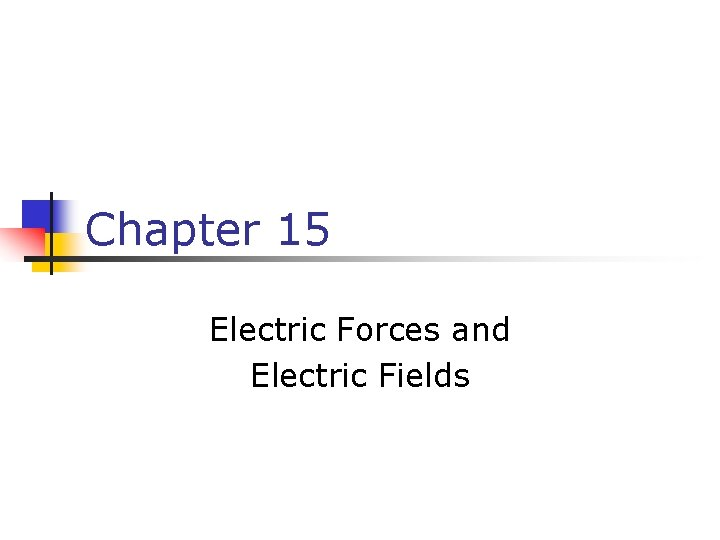Chapter 15 Electric Forces and Electric Fields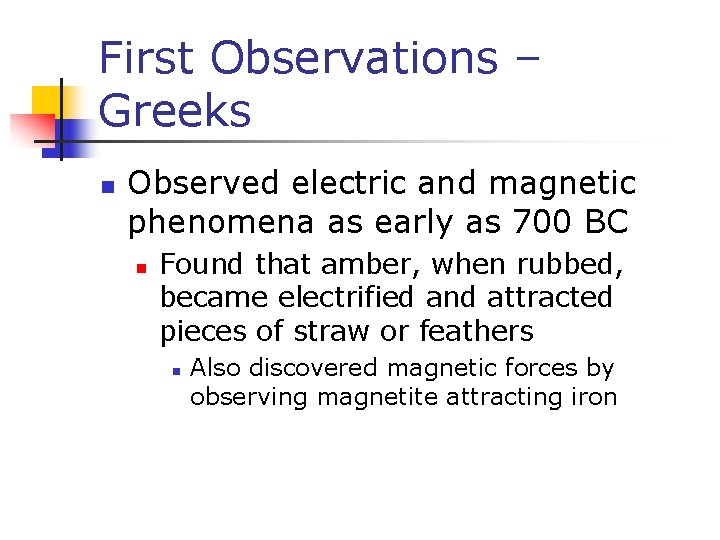First Observations – Greeks n Observed electric and magnetic phenomena as early as 700 BC n Found that amber, when rubbed, became electrified and attracted pieces of straw or feathers n Also discovered magnetic forces by observing magnetite attracting iron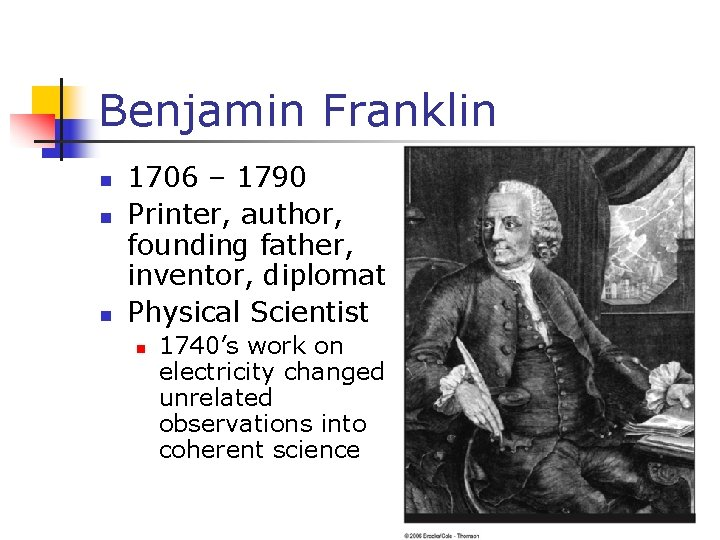Benjamin Franklin n 1706 – 1790 Printer, author, founding father, inventor, diplomat Physical Scientist n 1740’s work on electricity changed unrelated observations into coherent science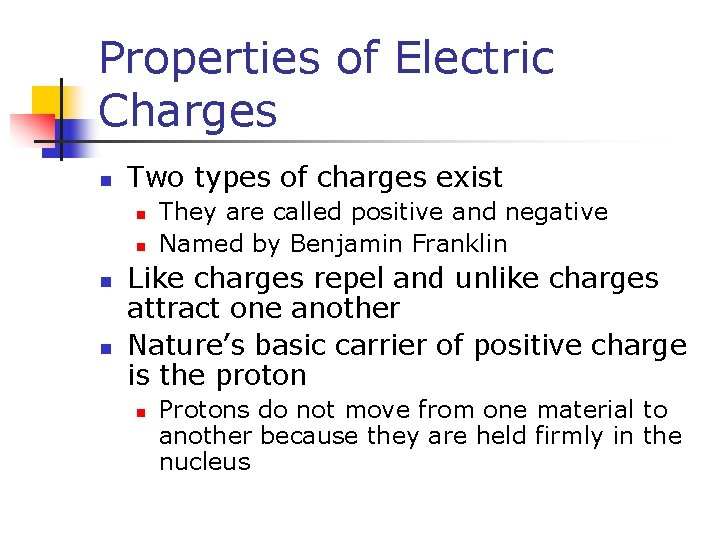Properties of Electric Charges n Two types of charges exist n n They are called positive and negative Named by Benjamin Franklin Like charges repel and unlike charges attract one another Nature’s basic carrier of positive charge is the proton n Protons do not move from one material to another because they are held firmly in the nucleusMore Properties of Charge n Nature’s basic carrier of negative charge is the electron n n Gaining or losing electrons is how an object becomes charged Electric charge is always conserved n n Charge is not created, only exchanged Objects become charged because negative charge is transferred from one object to anotherProperties of Charge, final n Charge is quantized n All charge is a multiple of a fundamental unit of charge, symbolized by e n n Quarks are the exception Electrons have a charge of –e Protons have a charge of +e The SI unit of charge is the Coulomb (C) n e = 1. 6 x 10 -19 C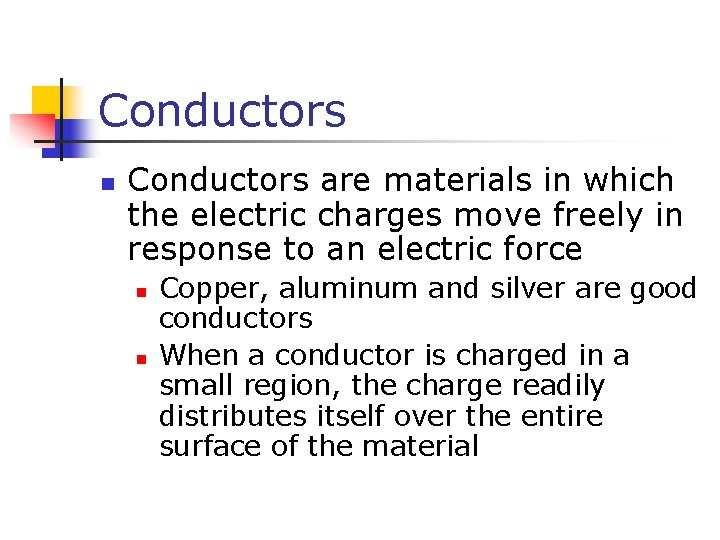Conductors n Conductors are materials in which the electric charges move freely in response to an electric force n n Copper, aluminum and silver are good conductors When a conductor is charged in a small region, the charge readily distributes itself over the entire surface of the materialInsulators n Insulators are materials in which electric charges do not move freely n n Glass and rubber are examples of insulators When insulators are charged by rubbing, only the rubbed area becomes charged n There is no tendency for the charge to move into other regions of the materialSemiconductors n n The characteristics of semiconductors are between those of insulators and conductors Silicon and germanium are examples of semiconductorsCharging by Conduction n n A charged object (the rod) is placed in contact with another object (the sphere) Some electrons on the rod can move to the sphere When the rod is removed, the sphere is left with a charge The object being charged is always left with a charge having the same sign as the object doing the chargingCharging by Induction n n When an object is connected to a conducting wire or pipe buried in the earth, it is said to be grounded A negatively charged rubber rod is brought near an uncharged sphereCharging by Induction, 2 n The charges in the sphere are redistributed n Some of the electrons in the sphere are repelled from the electrons in the rod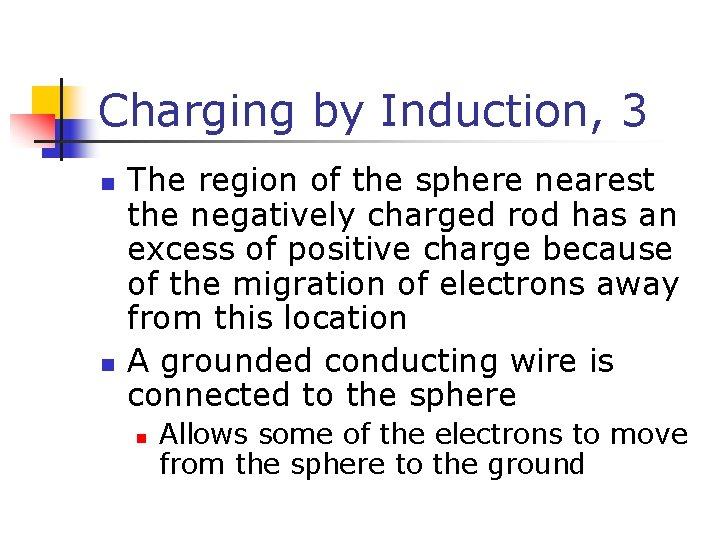Charging by Induction, 3 n n The region of the sphere nearest the negatively charged rod has an excess of positive charge because of the migration of electrons away from this location A grounded conducting wire is connected to the sphere n Allows some of the electrons to move from the sphere to the ground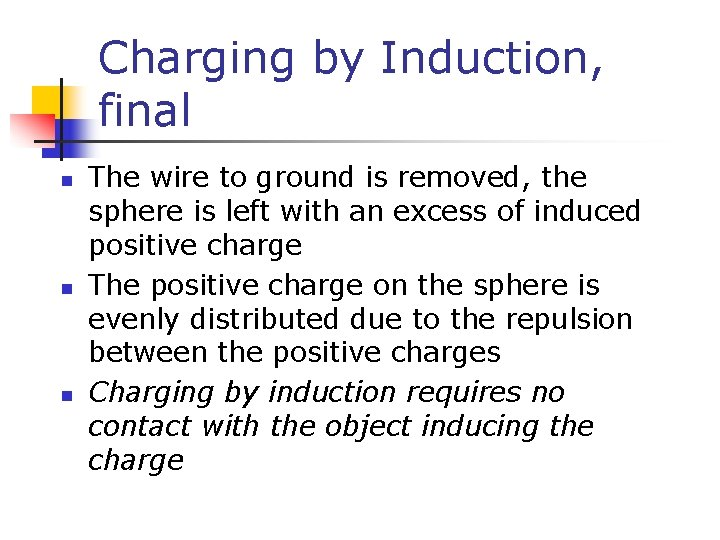Charging by Induction, final n n n The wire to ground is removed, the sphere is left with an excess of induced positive charge The positive charge on the sphere is evenly distributed due to the repulsion between the positive charges Charging by induction requires no contact with the object inducing the chargePolarization n n In most neutral atoms or molecules, the center of positive charge coincides with the center of negative charge In the presence of a charged object, these centers may separate slightly n n This results in more positive charge on one side of the molecule than on the other side This realignment of charge on the surface of an insulator is known as polarization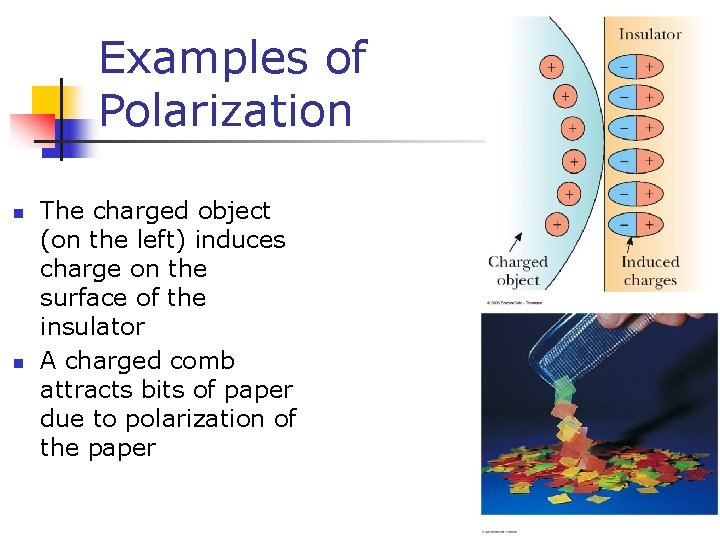Examples of Polarization n n The charged object (on the left) induces charge on the surface of the insulator A charged comb attracts bits of paper due to polarization of the paper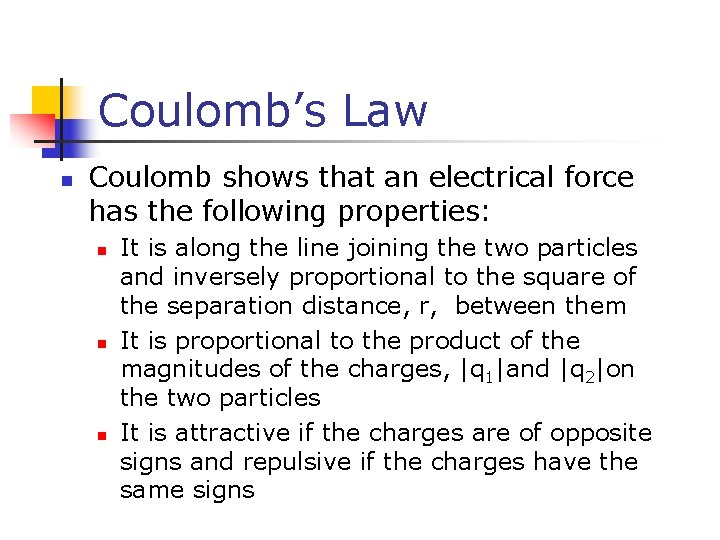Coulomb’s Law n Coulomb shows that an electrical force has the following properties: n n n It is along the line joining the two particles and inversely proportional to the square of the separation distance, r, between them It is proportional to the product of the magnitudes of the charges, |q 1|and |q 2|on the two particles It is attractive if the charges are of opposite signs and repulsive if the charges have the same signs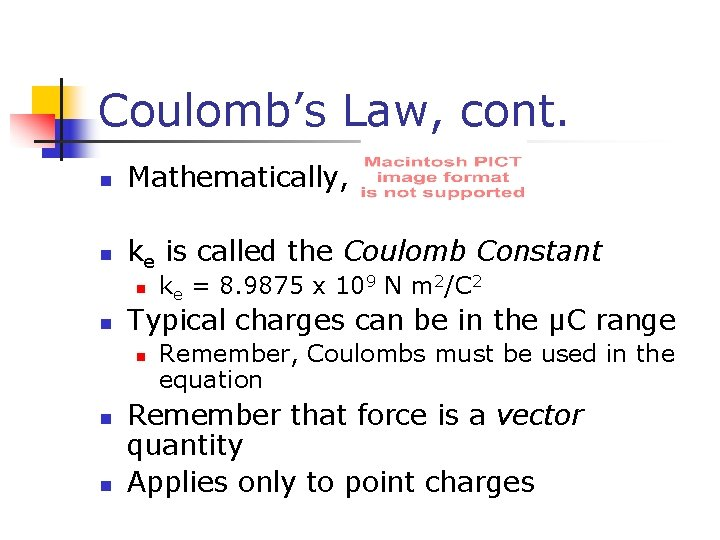Coulomb’s Law, cont. n Mathematically, n ke is called the Coulomb Constant n n Typical charges can be in the µC range n n n ke = 8. 9875 x 109 N m 2/C 2 Remember, Coulombs must be used in the equation Remember that force is a vector quantity Applies only to point chargesCharacteristics of ParticlesCharles Coulomb n n n 1736 – 1806 Studied electrostatics and magnetism Investigated strengths of materials n Identified forces acting on beams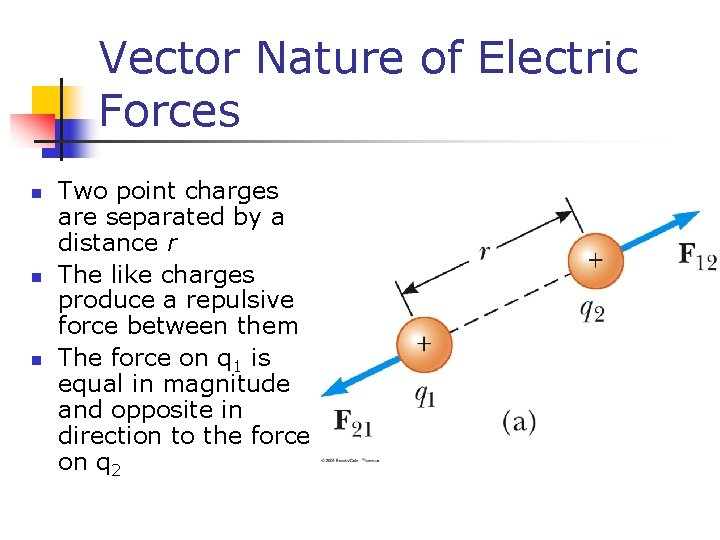Vector Nature of Electric Forces n n n Two point charges are separated by a distance r The like charges produce a repulsive force between them The force on q 1 is equal in magnitude and opposite in direction to the force on q 2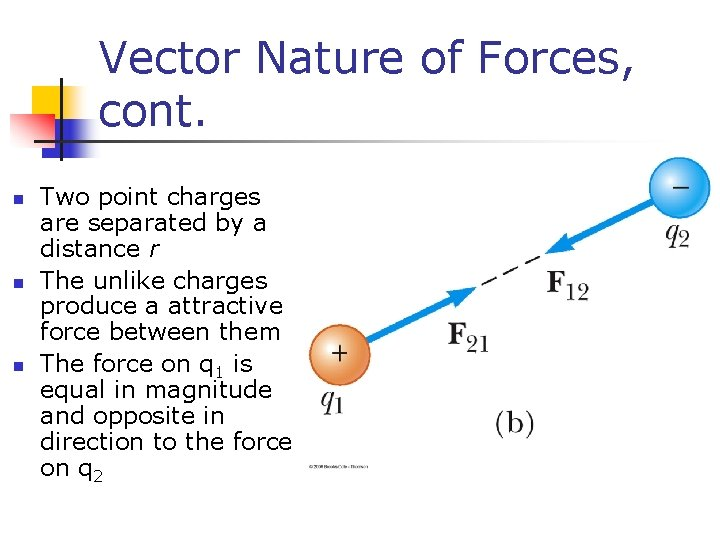Vector Nature of Forces, cont. n n n Two point charges are separated by a distance r The unlike charges produce a attractive force between them The force on q 1 is equal in magnitude and opposite in direction to the force on q 2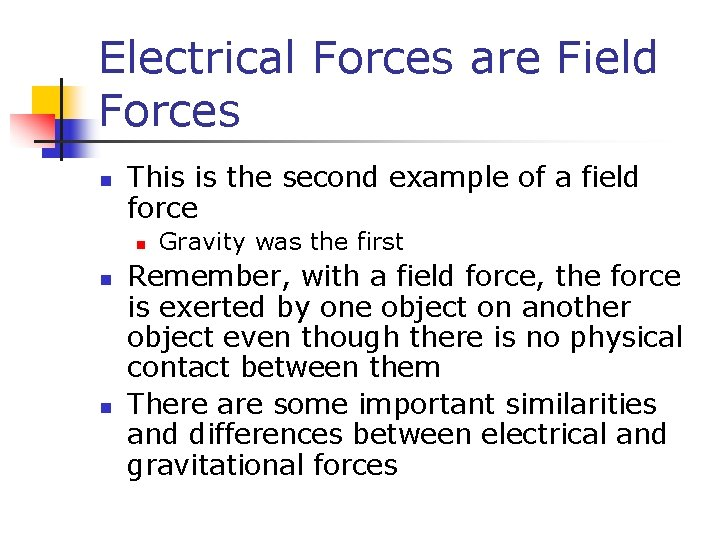Electrical Forces are Field Forces n This is the second example of a field force n n n Gravity was the first Remember, with a field force, the force is exerted by one object on another object even though there is no physical contact between them There are some important similarities and differences between electrical and gravitational forces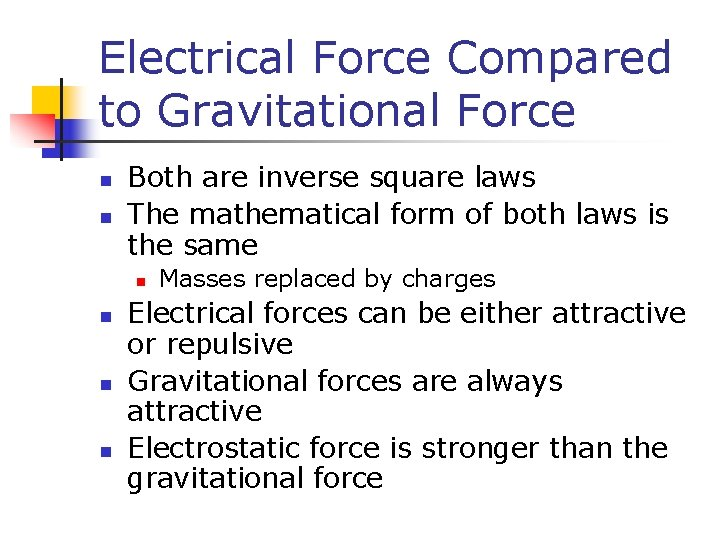Electrical Force Compared to Gravitational Force n n Both are inverse square laws The mathematical form of both laws is the same n n Masses replaced by charges Electrical forces can be either attractive or repulsive Gravitational forces are always attractive Electrostatic force is stronger than the gravitational forceThe Superposition Principle n The resultant force on any one charge equals the vector sum of the forces exerted by the other individual charges that are present. n Remember to add the forces as vectorsSuperposition Principle Example n n n The force exerted by q 1 on q 3 is The force exerted by q 2 on q 3 is The total force exerted on q 3 is the vector sum of and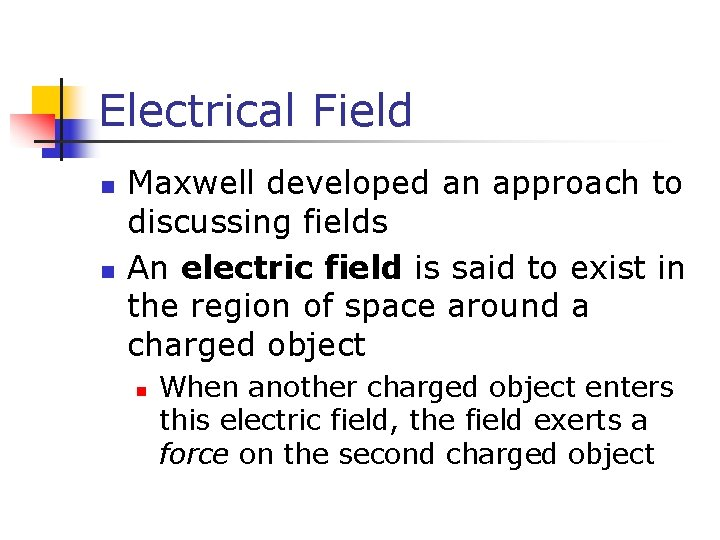Electrical Field n n Maxwell developed an approach to discussing fields An electric field is said to exist in the region of space around a charged object n When another charged object enters this electric field, the field exerts a force on the second charged object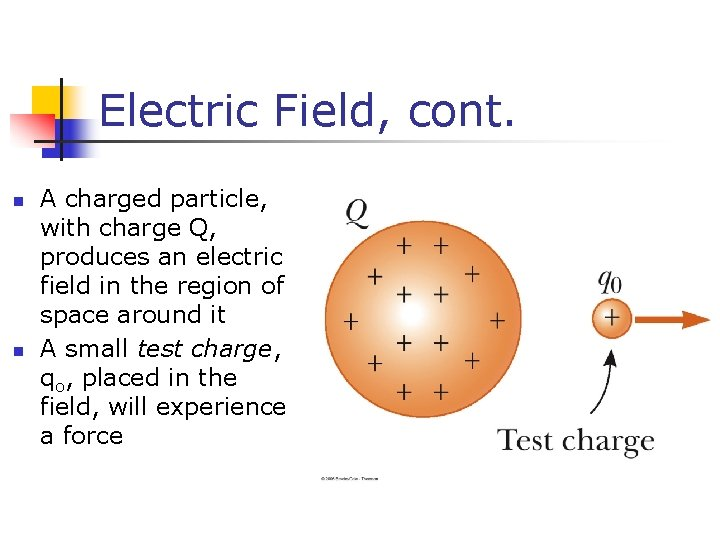Electric Field, cont. n n A charged particle, with charge Q, produces an electric field in the region of space around it A small test charge, qo, placed in the field, will experience a force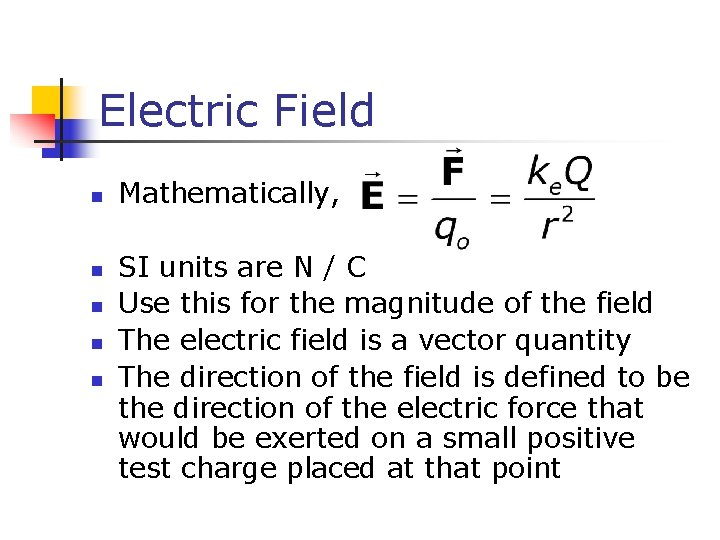Electric Field n n n Mathematically, SI units are N / C Use this for the magnitude of the field The electric field is a vector quantity The direction of the field is defined to be the direction of the electric force that would be exerted on a small positive test charge placed at that pointDirection of Electric Field n The electric field produced by a negative charge is directed toward the charge n A positive test charge would be attracted to the negative source charge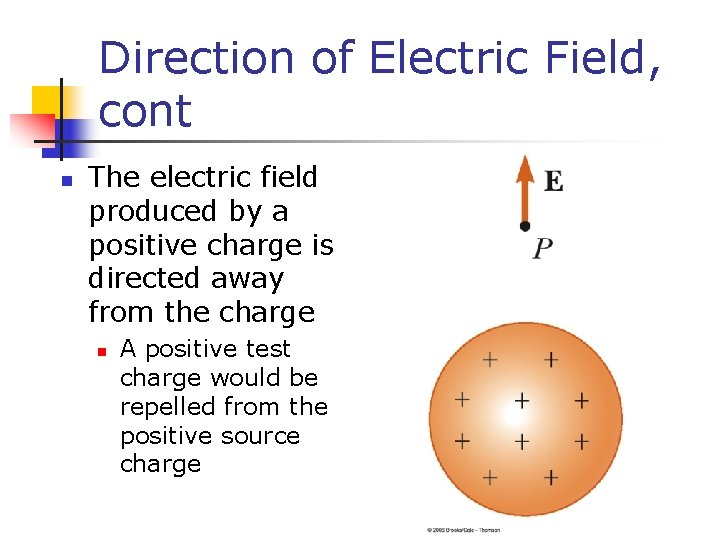Direction of Electric Field, cont n The electric field produced by a positive charge is directed away from the charge n A positive test charge would be repelled from the positive source charge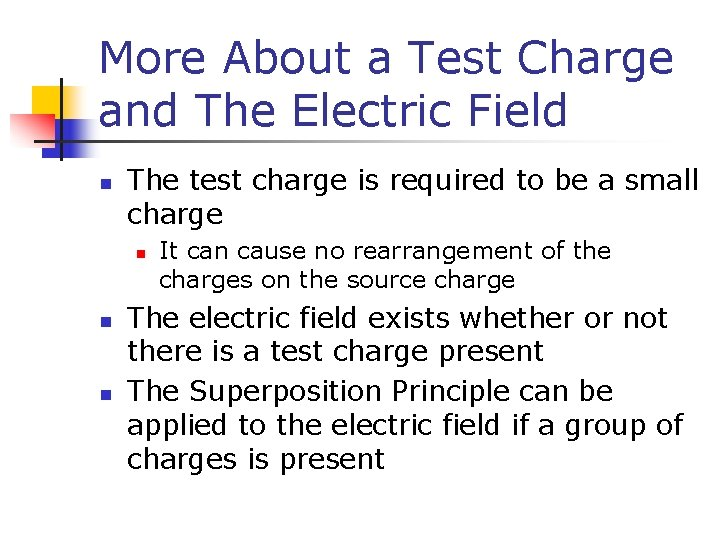More About a Test Charge and The Electric Field n The test charge is required to be a small charge n n n It can cause no rearrangement of the charges on the source charge The electric field exists whether or not there is a test charge present The Superposition Principle can be applied to the electric field if a group of charges is present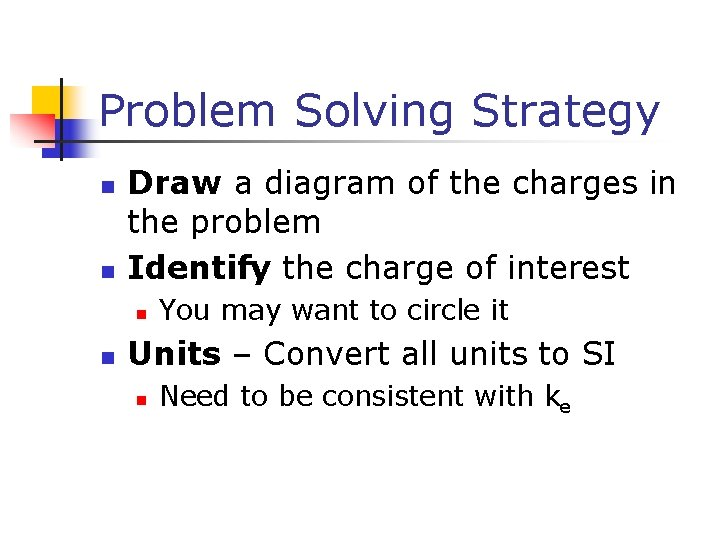Problem Solving Strategy n n Draw a diagram of the charges in the problem Identify the charge of interest n n You may want to circle it Units – Convert all units to SI n Need to be consistent with keProblem Solving Strategy, cont n Apply Coulomb’s Law n n n Sum all the x- and y- components n n For each charge, find the force on the charge of interest Determine the direction of the force This gives the x- and y-components of the resultant force Find the resultant force by using the Pythagorean theorem and trigProblem Solving Strategy, Electric Fields n Calculate Electric Fields of point charges n n n Use the equation to find the electric field due to the individual charges The direction is given by the direction of the force on a positive test charge The Superposition Principle can be applied if more than one charge is present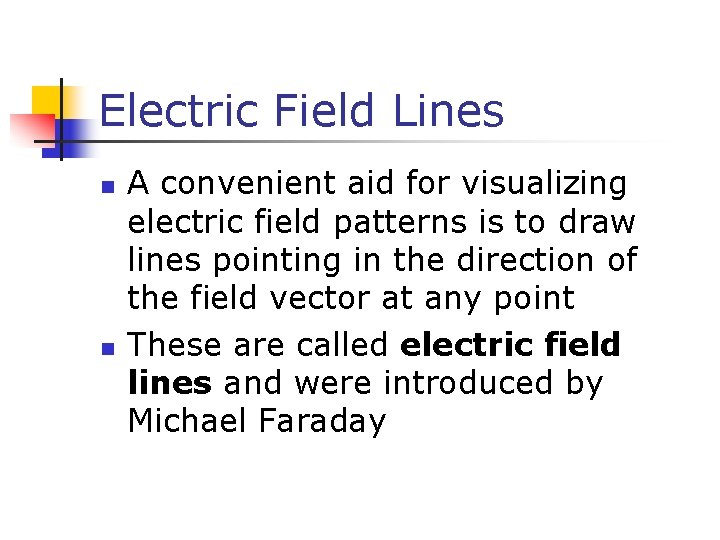Electric Field Lines n n A convenient aid for visualizing electric field patterns is to draw lines pointing in the direction of the field vector at any point These are called electric field lines and were introduced by Michael Faraday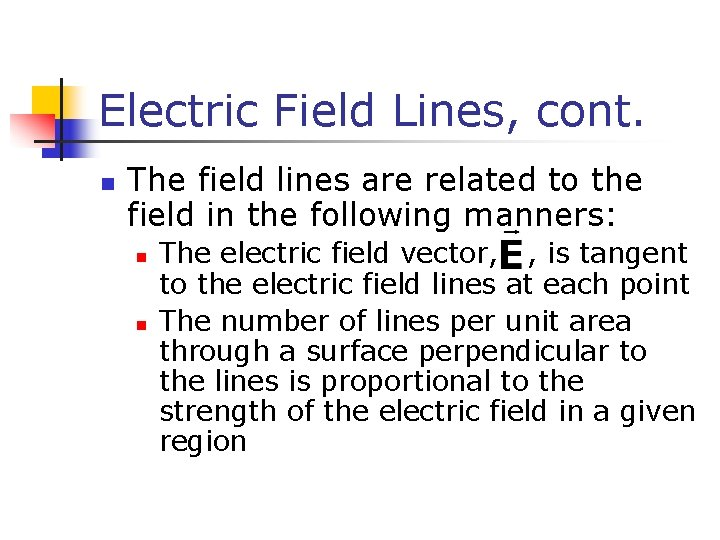Electric Field Lines, cont. n The field lines are related to the field in the following manners: n n The electric field vector, , is tangent to the electric field lines at each point The number of lines per unit area through a surface perpendicular to the lines is proportional to the strength of the electric field in a given region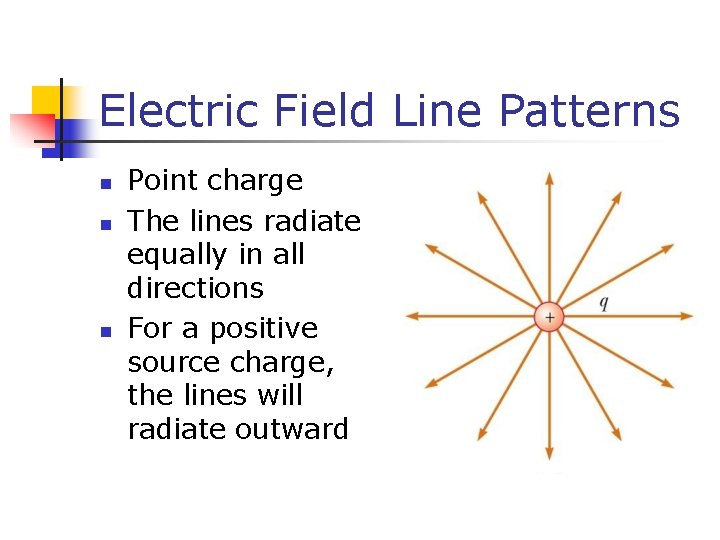Electric Field Line Patterns n n n Point charge The lines radiate equally in all directions For a positive source charge, the lines will radiate outward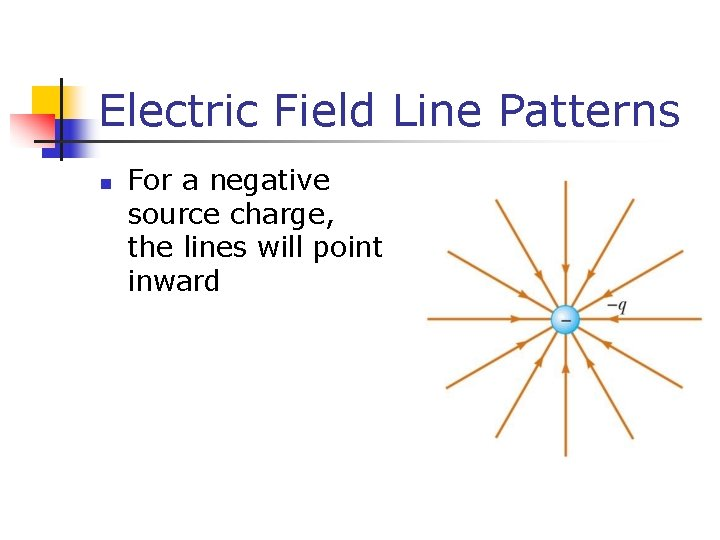Electric Field Line Patterns n For a negative source charge, the lines will point inwardElectric Field Line Patterns n n An electric dipole consists of two equal and opposite charges The high density of lines between the charges indicates the strong electric field in this region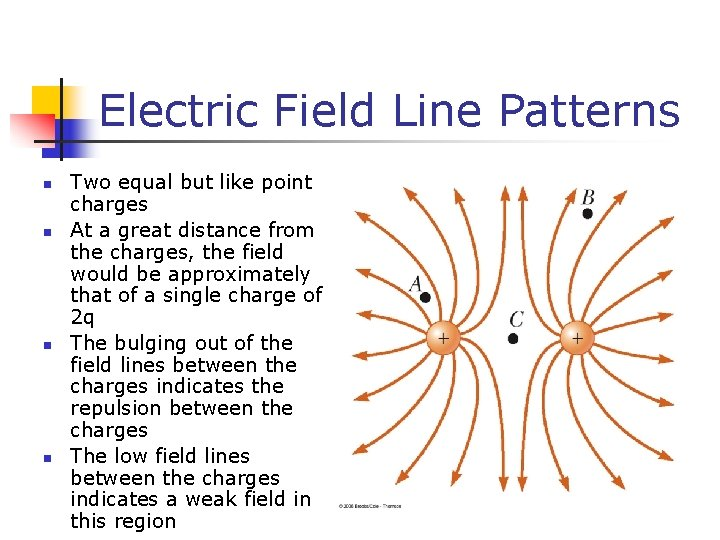Electric Field Line Patterns n n Two equal but like point charges At a great distance from the charges, the field would be approximately that of a single charge of 2 q The bulging out of the field lines between the charges indicates the repulsion between the charges The low field lines between the charges indicates a weak field in this region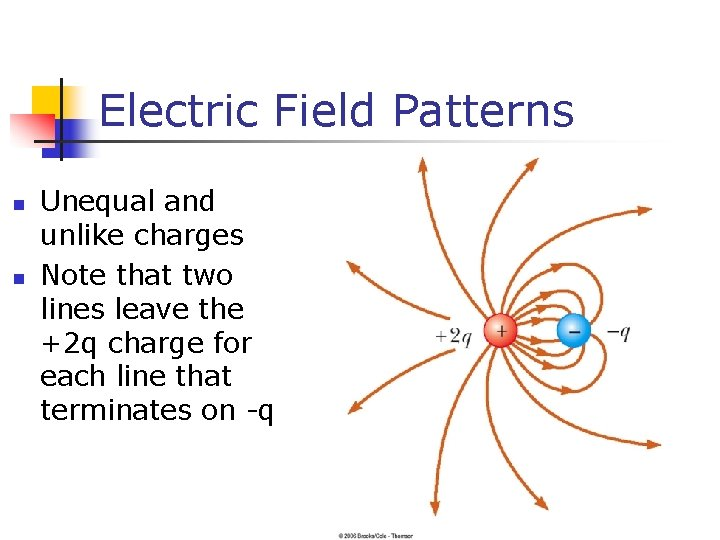Electric Field Patterns n n Unequal and unlike charges Note that two lines leave the +2 q charge for each line that terminates on -qRules for Drawing Electric Field Lines n The lines for a group of charges must begin on positive charges and end on negative charges n n n In the case of an excess of charge, some lines will begin or end infinitely far away The number of lines drawn leaving a positive charge or ending on a negative charge is proportional to the magnitude of the charge No two field lines can cross each otherConductors in Electrostatic Equilibrium n n When no net motion of charge occurs within a conductor, the conductor is said to be in electrostatic equilibrium An isolated conductor has the following properties: n n The electric field is zero everywhere inside the conducting material Any excess charge on an isolated conductor resides entirely on its surface The electric field just outside a charged conductor is perpendicular to the conductor’s surface On an irregularly shaped conductor, the charge accumulates at locations where the radius of curvature of the surface is smallest (that is, at sharp points)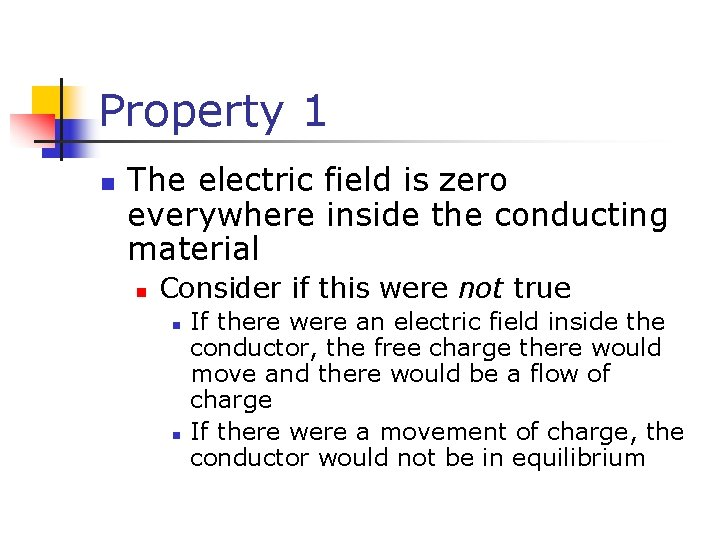Property 1 n The electric field is zero everywhere inside the conducting material n Consider if this were not true n n If there were an electric field inside the conductor, the free charge there would move and there would be a flow of charge If there were a movement of charge, the conductor would not be in equilibriumProperty 2 n Any excess charge on an isolated conductor resides entirely on its surface n n A direct result of the 1/r 2 repulsion between like charges in Coulomb’s Law If some excess of charge could be placed inside the conductor, the repulsive forces would push them as far apart as possible, causing them to migrate to the surface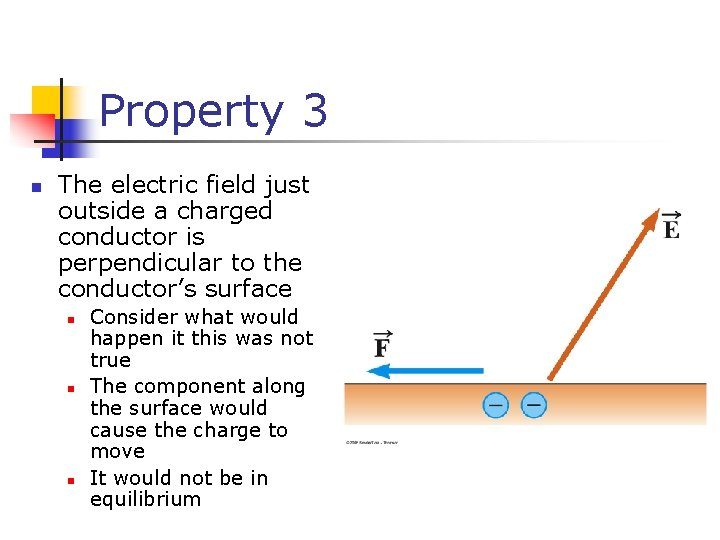Property 3 n The electric field just outside a charged conductor is perpendicular to the conductor’s surface n n n Consider what would happen it this was not true The component along the surface would cause the charge to move It would not be in equilibrium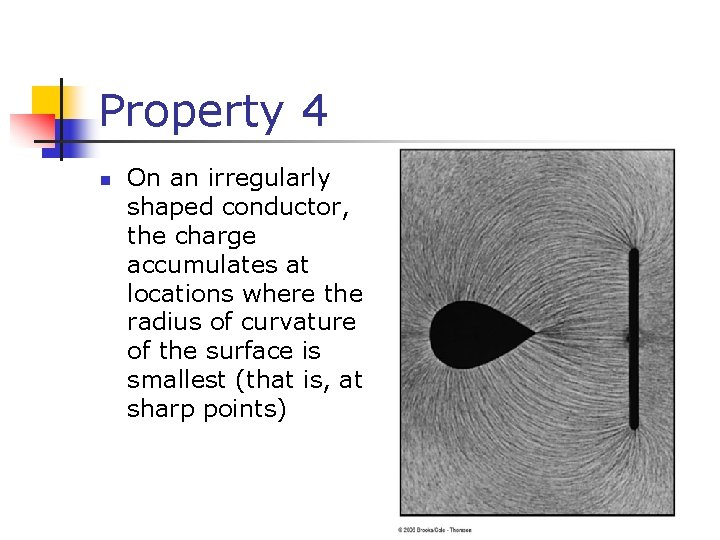Property 4 n On an irregularly shaped conductor, the charge accumulates at locations where the radius of curvature of the surface is smallest (that is, at sharp points)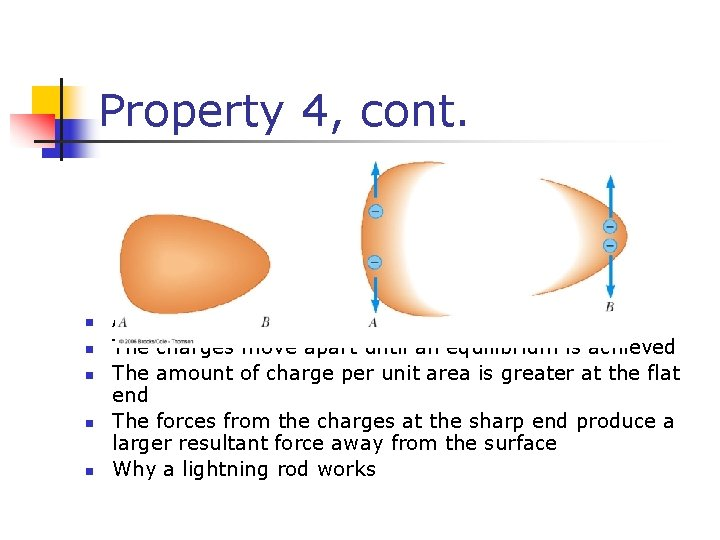Property 4, cont. n n n Any excess charge moves to its surface The charges move apart until an equilibrium is achieved The amount of charge per unit area is greater at the flat end The forces from the charges at the sharp end produce a larger resultant force away from the surface Why a lightning rod works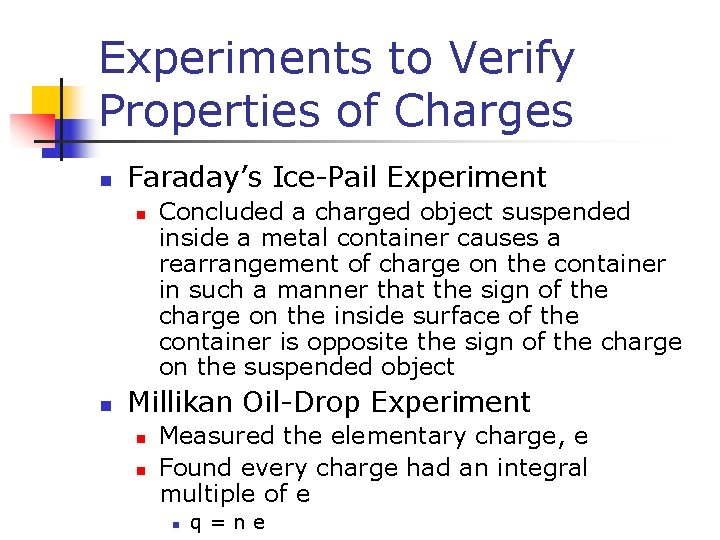Experiments to Verify Properties of Charges n Faraday’s Ice-Pail Experiment n n Concluded a charged object suspended inside a metal container causes a rearrangement of charge on the container in such a manner that the sign of the charge on the inside surface of the container is opposite the sign of the charge on the suspended object Millikan Oil-Drop Experiment n n Measured the elementary charge, e Found every charge had an integral multiple of e n q=neVan de Graaff Generator n n n An electrostatic generator designed and built by Robert J. Van de Graaff in 1929 Charge is transferred to the dome by means of a rotating belt Eventually an electrostatic discharge takes place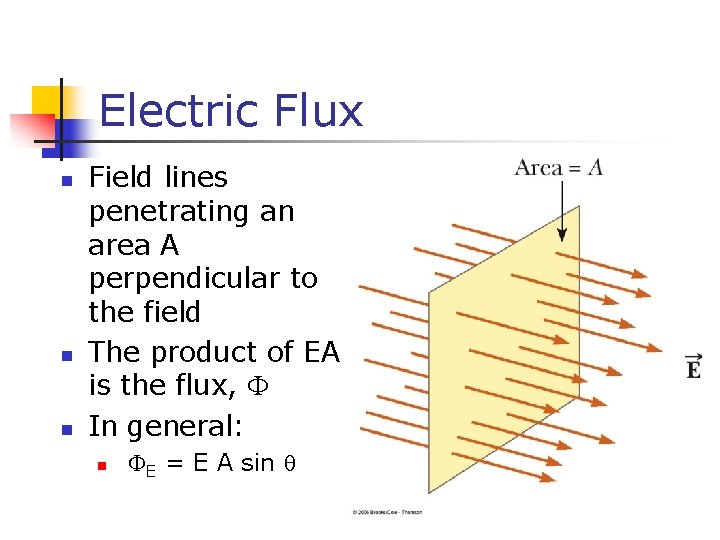Electric Flux n n n Field lines penetrating an area A perpendicular to the field The product of EA is the flux, In general: n E = E A sinElectric Flux, cont. n E = E A sin n n The perpendicular to the area A is at an angle to the field When the area is constructed such that a closed surface is formed, use the convention that flux lines passing into the interior of the volume are negative and those passing out of the interior of the volume are positive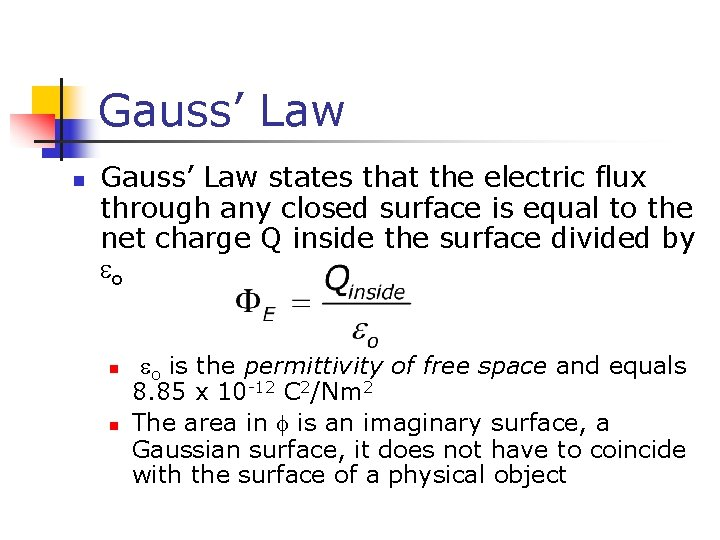Gauss’ Law n Gauss’ Law states that the electric flux through any closed surface is equal to the net charge Q inside the surface divided by o n n o is the permittivity of free space and equals 8. 85 x 10 -12 C 2/Nm 2 The area in is an imaginary surface, a Gaussian surface, it does not have to coincide with the surface of a physical object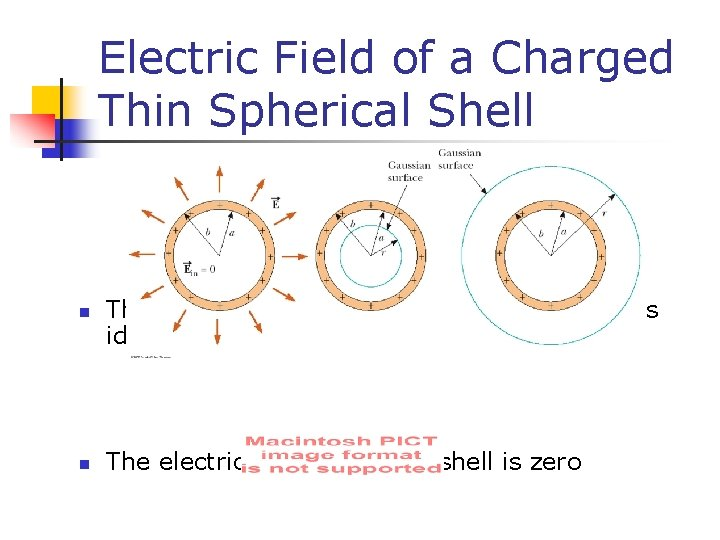Electric Field of a Charged Thin Spherical Shell n n The calculation of the field outside the shell is identical to that of a point charge The electric field inside the shell is zeroElectric Field of a Nonconducting Plane Sheet of Charge n n Use a cylindrical Gaussian surface The flux through the ends is EA, there is no field through the curved part of the surface The total charge is Q = A Note, the field is uniform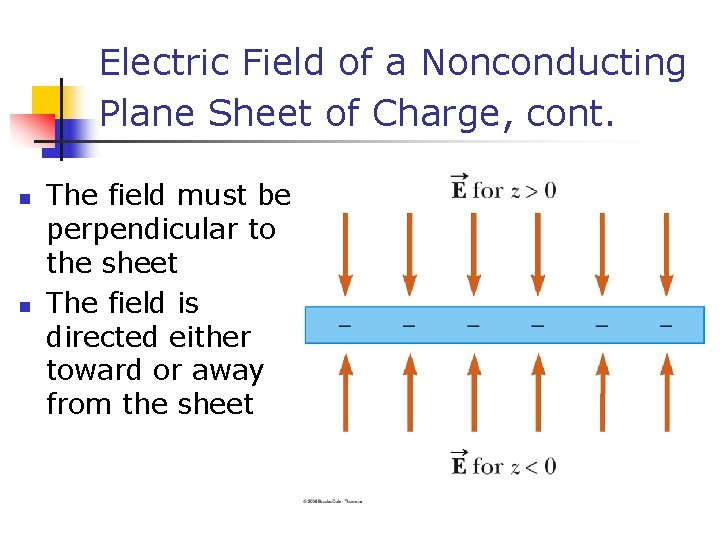Electric Field of a Nonconducting Plane Sheet of Charge, cont. n n The field must be perpendicular to the sheet The field is directed either toward or away from the sheet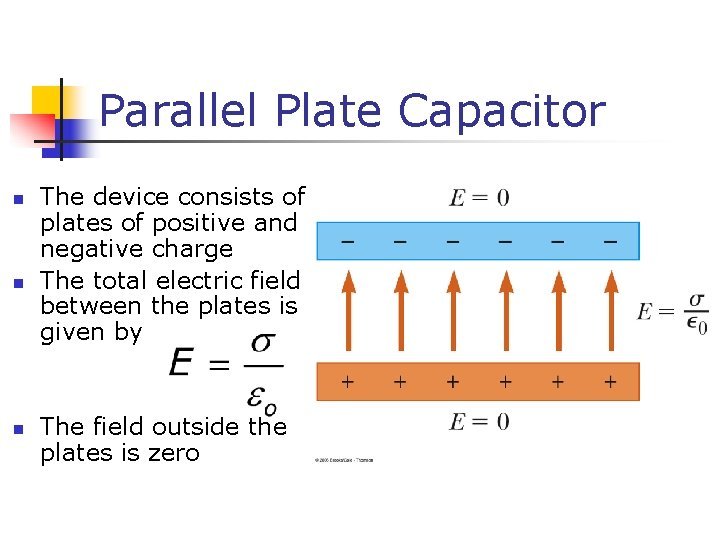Parallel Plate Capacitor n n n The device consists of plates of positive and negative charge The total electric field between the plates is given by The field outside the plates is zero